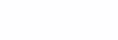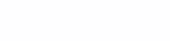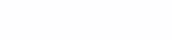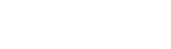# Risks and Caveats When Applying Principal Component Method to Supervised Learning Problems

Translation of the article was prepared on the eve of the start Basic Machine Learning Course

### High-dimensional space and its curse

The curse of dimensionality is a serious problem when dealing with real datasets, which tend to be multidimensional. As the dimension of the feature space increases, the number of configurations can grow exponentially, and, as a result, the number of configurations covered by observation decreases.

In such a case, the principal component analysis (PCA) will play an important role, effectively downsizing the data while retaining as much variation as possible in the dataset.

Let’s take a quick look at the essence of principal component analysis before diving into the problem.

### Principal Component Method – definition

The main idea behind principal component analysis is to reduce the dimension of a dataset that is composed of a large number of interrelated variables, while maintaining the maximum diversity that is present in the dataset.

We define a symmetric matrix AND,Where X – matrix mxn independent variables, where m Is the number of columns, and n Is the number of data points. The matrix AND can be decomposed as follows:Where D Is a diagonal matrix, and E – matrix of eigenvectors ANDarranged in columns.

Main components X Are own vectors XXT, which suggests that the direction of the eigenvectors / principal components depends on the variation of the independent variable (X)

Why is the reckless application of principal component analysis the bane of supervised learning problems?

The literature often mentions the use of the principal component analysis in regression, as well as in multicollinearity problems. However, along with the use of regression on principal components, there were many misconceptions about the explainability of the response variable by principal components and the order of their importance.

A common misconception, which has been encountered several times in various articles and books, is that in a supervised learning environment with principal component regression, principal components of the independent variable with small eigenvalues ​​will not play an important role in explaining the response variable, which leads us to the purpose of this article. The idea is that components with small eigenvalues ​​can be just as important, or even much more important, than basic components with large eigenvalues ​​in explaining the response variable.

Below I will list a few examples of publications that I mentioned:

… Mansfield et al. (1977, p. 38) suggest that if only low variance components are removed, then the regression does not lose much predictive power.
… In Ganst and Mason (1980), 12 pages are devoted to principal component regression, and much of the discussion suggests that the removal of principal components is based solely on their variances. (pp. 327–328).
… Mosteller and Türki (1977, pp. 397–398) also argue that low variance components are unlikely to be important in regression, apparently because nature “Tricky”, but not “Uniform”
… Hawking (1976, p. 31) is even more restrictive in defining the principle of conservation of principal components in regression based on variance.

### Theoretical explanation and understanding

First, let’s get the correct mathematical justification for the above hypothesis, and then give a little explanation for a better understanding using geometric visualization and modeling.

Let’s say
Y – response variable,
X – Feature space matrix
Z – Standardized version X

Let it go $inline \lambda ₁\ge \lambda ₂>\dots . \ge \lambda p inline$ will be eigenvalues ZTZ (correlation matrix), and V – the corresponding eigenvectors, then in W = ZV, columns in W will represent the main components Z… The standard method used for principal component regression is to regress the first m principal components on Y, and the problem can be represented through the theorem below and its explanation …

## Theorem:

Let be W = (W₁,…, Wp) – own vectors X… Now let’s look at the regression model:If the true vector of regression coefficients β co-directional with the j-th eigenvector ZTZ, then in regression Y on W, jth main component Wⱼ will contribute to learning, while the rest will not contribute in principle.

Evidence: Let be V = (V₁,…, Vp) – matrix of eigenvectors ZTZ… ThenAswhereexpression regression coefficients.

If a β aligned with j-th eigenvector Vⱼthen Vⱼ = aβwhere a – nonzero scalar value. Hence, θj = Vⱼᵀβ = aβᵀβ and θᴋ = Vᴋᵀβ = 0, where k ≠ j… Thus, the regression coefficient θᴋ corresponding to Wᴋ is zero, for k ≠ j, respectively,Since the variable Wᴋ does not reduce the sum of squares, if its regression coefficient is 0, then Wj will provide the main contribution, while the other main components will not contribute at all.

### Geometric significance and modeling

Now let’s simulate and get a geometric representation of the above mathematical calculations. The explanation is illustrated by modeling a two-dimensional feature space (X) and one response variable so that the hypothesis can be easily understood visually.Figure 1: One-dimensional and two-dimensional plots for considered variables X1 and X2

In the first stage of modeling, the feature space was modeled using a multivariate normal distribution with a very high correlation between variables and principal components.Figure 2: Heat Map Correlation for PC1 and PC2 (Principal Components)

It is very clearly seen from the graph that there is no correlation between the principal components. At the second step, the values ​​of the response variable Y are modeled so that the direction of the Y coefficient of the principal components coincides with the direction of the second principal component.After receiving the response variable, the correlation matrix will look something like this.Figure 3: Heat Map for Variable Y and PC1 and PC2.

The graph clearly shows that between Y and PC2 the correlation is higher than between Y and PC1, which confirms our hypothesis.Figure 4: Feature space variance explained by PC1 and PC2.

Since the figure shows that PC1 explains 95% of variance X, then according to the logic stated above, we should completely ignore PC2 with regression.

So let’s follow it and see what happens!Figure 5. Result of regression with Y and PC1.

Thus equal to 0, suggests that despite the fact that PC1 gives 95% variance X, it still doesn’t explain the response variable.

Now let’s do the same with PC2, which explains only 5% of the variance X, and see what comes of it.Figure 6: Result of regression with Y and PC2.

Yuhu! Just look at what happened: the main component that explained five% variance X, gave 72% variance Y… There are also real examples to support such situations:

 Smith and Campbell (1980) gave an example from chemical engineering where there were 9 regressive variables, and when the variance of the eighth principal component was 0.06% of the total variance, which would not be taken into account due to the above logic.
 A second example was provided by Kung and Sharif (1980). In a study of predicting the start date of monsoons using ten meteorological variables, only the eighth, second and tenth components were significant. This example shows that even the principal component with the smallest eigenvalue will be the third most significant in terms of explaining the variability of the response variable.

### Output

The above examples show that it is inappropriate to remove principal components with small eigenvalues, since they affect only the explainability in the feature space, but not the response variable. Therefore, all the components need to be preserved in supervised dimension reduction techniques, such as partial least squares regression and least angle regression, which we will talk about in future articles.

#### Sources:

 Jolliffe, Ian T. “A Note on the Use of Principal Components in Regression.” Journal of the Royal Statistical Society. Series C (Applied Statistics), vol. 31, no. 3, 1982, pp. 300-303. JSTOR, www.jstor.org/stable/2348005
 Hadi, Ali S., and Robert F. Ling. “Some Cautionary Notes on the Use of Principal Components Regression.” The American Statistician, vol. 52, no. 1, 1998, pp. 15-19. JSTOR, www.jstor.org/stable/2685559
 HAWKINS, DM (1973). On the investigation of alternative regressions by principal component analysis. Appl. Statist., 22, 275-286
 MANSFIELD, ER, WEBSTER, JT and GUNST, RF (1977). An analytic variable selection technique for principal component regression. Appl. Statist., 26, 34-40.
 MOSTELLER, F. and TUKEY, JW (1977). Data Analysis and Regression: A Second Course in Statistics. Reading, Mass .: Addison-Wesley
 GUNST, RF and MASON, RL (1980). Regression Analysis and its Application: A Data-oriented Approach. New York: Marcel Dekker.
 JEFFERS, JNR (1967). Two case studies in the application of principal component analysis. Appl. Statist. 16, 225-236. (1981). Investigation of alternative regressions: some practical examples. The Statistician, 30, 79–88.
 KENDALL, MG (1957). A Course in Multivariate Analysis. London: Griffin.